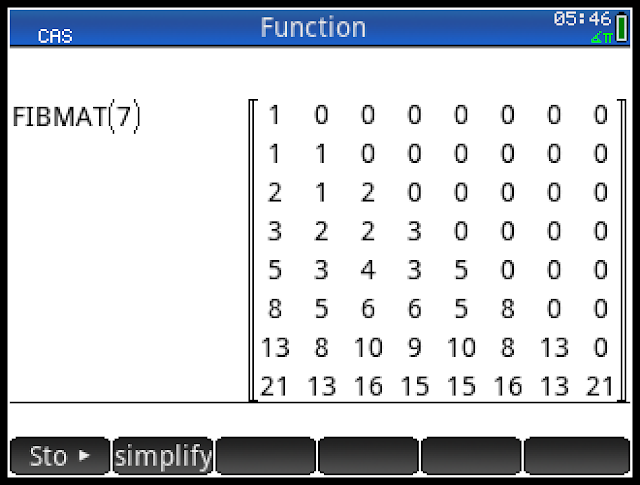Fibonacci Triangle Matrices
12-20-2018, 02:29 PM
Post: #1Eddie W. ShoreSenior Member Posts: 1,014 Joined: Dec 2013
Fibonacci Triangle Matrices
Original blog post: https://edspi31415.blogspot.com/2018/12/...nacci.html

This program is the request of John Cvetan. I thank you for your suggestion.

To generate the Fibonacci triangle,

1. Let r the row and c be the column with

f_0,0 = 1
f_1,0 = 1
f_1,1 = 1
f_2,1 = 1

2. Each row will be determined by adding the last two terms going diagonally. You can use one of two formulas:

f_r,c = f_r-1,c + f_r-2,c

f_r,c = f_r-1,c-1 + f_r-1,c-2

The Program FIBMAT

FIBMAT generates a Fibonacci triangle in matrix form. It's the result is a triangle that is "tilted". n will need to be 3 or greater.

Code:
EXPORT FIBMAT(n) BEGIN // Fibonacci "triangle" in // matrix form // 2018-12-17 EWS LOCAL M1,k; M1:=MAKEMAT(0,n+1,n+1); M1(1,1):=1; M1(2,1):=1; M1(2,2):=1; FOR k FROM 3 TO n+1 DO M1(k):=row(M1,k-1)+row(M1,k-2); M1(k,k):=M1(k-1,k-1)+M1(k-2,k-2); END; RETURN M1; END;

The Program FIBTRI

This is a visual program for Fibonacci Triangle.

FIBTRI(n) generates a visual Fibonacci Triangle - although I don't recommend going beyond 12 rows due to the constraints of the screen. I used the small font for the rows.

HP Prime Program FIBTRI
Code:
 EXPORT FIBTRI(n) BEGIN // Fibonacci triangle // 2018-12-17 EWS LOCAL M1,k; M1:=MAKEMAT(0,n+1,n+1); M1(1,1):=1; M1(2,1):=1; M1(2,2):=1; FOR k FROM 3 TO n+1 DO M1(k):=row(M1,k-1)+row(M1,k-2); M1(k,k):=M1(k-1,k-1)+M1(k-2,k-2); END; RECT(); LOCAL s; FOR k FROM 1 TO n+1 DO s:=STRING(SUB(row(M1,k),1,k)); IF k≤6 THEN TEXTOUT_P(s, 140-5.5*(k-1),(k-1)*15,2); END; IF k>6 AND k≤11 THEN TEXTOUT_P(s, 140-8*(k-1),(k-1)*15,2); END; IF k>11 THEN TEXTOUT_P(s, 140-11.5*(k-1),(k-1)*15,2); END; END; WAIT(0); END;
Source:

Hosoya, Haruo. "Fibonacci Triangle" Ochanomizu University, Tokyo, Japan. 1976. https://www.fq.math.ca/Scanned/14-2/hosoya.pdf
12-21-2018, 04:01 PM
Post: #2
 John KeithSenior Member Posts: 459 Joined: Dec 2013
RE: Fibonacci Triangle Matrices
Thanks for posting this, I was not aware of the Fibonacci triangle until now. More info can be found here.

Computation can be sped up significantly by taking advantage of the symmetry of the triangle. Only the first CEIL(n/2) values in row n need be calculated. Then copy the first FLOOR(n/2) values, reversed, to the end of the row. Not really needed in this example but useful for other triangles whose values are harder to calculate.
12-22-2018, 03:00 AM
Post: #3Eddie W. ShoreSenior Member Posts: 1,014 Joined: Dec 2013
RE: Fibonacci Triangle Matrices
Is anyone getting errors running either FIBTRI or FIBMAT? Please let me know whether the program runs OK or not. Thanks!
12-22-2018, 07:07 AM
Post: #4
 DA74254Member Posts: 130 Joined: Sep 2017
RE: Fibonacci Triangle Matrices
(12-22-2018 03:00 AM)Eddie W. Shore Wrote:  Is anyone getting errors running either FIBTRI or FIBMAT? Please let me know whether the program runs OK or not. Thanks!

It won't run as program or in CAS. Both functions runs OK in Home/RPN

Esben
28s, 35s, 49G+, 50G, Prime G1 HW A, Prime G2 HW D, SwissMicros DM42
Elektronika MK-52 & MK-61
12-22-2018, 03:01 PM
Post: #5
 Eric RechlinMember Posts: 219 Joined: Dec 2013
RE: Fibonacci Triangle Matrices
I am seeing the same behavior. It runs from Home but not from the Programs menu.

Interestingly, when I try to debug them from the Programs menu, I was able to step through and debug both FIBTRI and FIBMAT just once each, but then subsequent attempts at debugging them failed with the same "Bad argument type" error on the first line inside the first FOR loop (where the output of the sum of the calls to "row" is assigned to the row of the M1 matrix).

I'm wondering if this is a bug in the Virtual Calculator on some systems. I am seeing this in the 64-bit version of the 2018-10-16 release on Windows 10, though I saw the same error on the 32-bit version of the 2018-07-06 release also.
12-22-2018, 05:31 PM (This post was last modified: 12-22-2018 05:47 PM by Albert Chan.)
Post: #6
 Albert ChanSenior Member Posts: 727 Joined: Jul 2018
RE: Fibonacci Triangle Matrices
Tried FIBMAT code with XCas 1.4.9-57 (win32). It seems there is such a thing as "too flexible".
Maple and Mupad program style work as expected. However ...

XCas style assumed row(M1, 0) as first row, thus will not work unless change the code:

Replace row(M1, r) ==> M1(r)

Perhaps this is the reason for "Bad argument type" error.
12-22-2018, 06:33 PM
Post: #7compsystemsSenior Member Posts: 1,183 Joined: Dec 2013
RE: Fibonacci Triangle Matrices
The FIBMAT() current code only works in numerical mode (HOME), but it must be taken into account that if the index variable that can only be changed in CAS mode, is in 'index': = 0, it affects the output of the function

test
Steps:
1: Press [CAS]
2: index:=0 [↵] returns "[] index start 0"
3: then Press [HOME]
4: FIBMAT(7) [↵]
[[1,0,0,0,0,0,0,0],[1,1,0,0,0,0,0,0],[1,1,2,0,0,0,0,0],[1,1,2,3,0,0,0,0],[1,1,2,3,5,0,0,0],[1,1,2,3,5,8,0,0],[1,1,2,3,5,8,13,0],[1,1,2,3,5,8,13,21]]

5: Press [CAS]
6: index:=1 [↵] returns "[] index start 1"
7: then Press [HOME]
8: FIBMAT(7) [↵]
[[1,0,0,0,0,0,0,0],[1,1,0,0,0,0,0,0],[2,1,2,0,0,0,0,0],[3,2,2,3,0,0,0,0],[5,3,4,3,5,0,0,0],[8,5,6,6,5,8,0,0],[13,8,10,9,10,8,13,0],[21,13,16,15,15,16,13,21]]I try to change the value of the internal variable within the code but it fails.
CAS.index:=1;

PHP Code:
EXPORT FIBMAT(n)BEGIN// Fibonacci "triangle" in// matrix form// 2018-12-17 EWSLOCAL M1,k;CAS.index:=1;M1:=MAKEMAT(0,n+1,n+1);M1(1,1):=1;M1(2,1):=1;M1(2,2):=1;FOR k FROM 3 TO n+1 DOM1(k):=row(M1,k-1)+row(M1,k-2);M1(k,k):=M1(k-1,k-1)+M1(k-2,k-2);END;RETURN M1;END;

LO SUFICIENTEMENTE BUENO ES ENEMIGO DE LA EXCELENCIA.
12-23-2018, 09:33 PM
Post: #8Eddie W. ShoreSenior Member Posts: 1,014 Joined: Dec 2013
RE: Fibonacci Triangle Matrices
Here's an alternate code for Fibonacci Triangle Matrices. The row command has been eliminated and I used a second For loop in its place.

Code:
EXPORT FIBMATALT(n) BEGIN // 2018-12-23 EWS // Fibonacci Matrix Alternate // matrix form // This version does not have the // row function. LOCAL M1,k,j; M1:=MAKEMAT(0,n+1,n+1); M1(1,1):=1; M1(2,1):=1; M1(2,2):=1; FOR k FROM 3 TO n+1 DO FOR j FROM 1 TO n DO M1(k,j):=M1(k-2,j)+M1(k-1,j); END; M1(k,k):=M1(k-1,k-1)+M1(k-2,k-2); END; RETURN M1; END;
12-23-2018, 09:59 PM (This post was last modified: 12-23-2018 10:00 PM by pier4r.)
Post: #9
 pier4rSenior Member Posts: 2,016 Joined: Nov 2014
RE: Fibonacci Triangle Matrices
Side note. From the link posted by eddie.

https://www.fq.math.ca/Scanned/ this is the fibonacci quarterly!

Wikis are great, Contribute :)
 « Next Oldest | Next Newest »

User(s) browsing this thread: 1 Guest(s)